Transient Analysis of Ocular Drug Delivery - Zero Volume Effect

by

J. C. Keisterx,1, P.S. Heidmann2 and P.J. Missel3

1 - 3M Pharmaceuticals, 3M Center, Bldg 270-4S-02, St. Paul, MN 55144-1000

(612)-733-0171;  FAX (612)-736-3974; jckeister@mmm.com

2 - Computing Devices International, BLCS2X, 8800 Queen Ave. S. Bloomington, MN   55431

3 - Alcon Labs, Inc., 6201 S. Freeway, Ft. Worth, TX 76134-2099

Abstract

Dose volume reduction is one method for reducing the nonproductive loss of ophthalmic drugs caused by premature drainage from the precorneal area.  A new mathematical method is presented for calculating the bioavailability enhancement achieved by the dose volume reduction method. This new model suggests that the steady-state assumption used in a previous paper overestimated the bioavailability enhancement, depending upon physical factors such as distribution and diffusion coefficients of the drug in tissue and solution.  The analysis shows that transient effects can make a difference up to a complete elimination of the zero volume effect in those cases where the distribution coefficient is large and the permeability coefficient is small (i.e., for large, lipophilic molecules).

Introduction and Background

In a previous paper, an attempt was made to establish the theoretical dependence of ocular bioavailability on the volume of the dose from an aqueous ophthalmic solution. The cul-de-sac volume, tear turnover rate and excess volume removal rate constant were taken from the literature,.  The analysis suggested that for drugs having a low permeability coefficient, up to a four-fold increase in drug bioavailability might be possible upon reducing the dose volume from 30 microliters to zero volume at constant dose.  As drug permeability increases, this benefit in bioavailability from reduced volume diminishes appreciably.

The original work presumed steady state diffusion loss of drug across the cornea, a valid assumption provided the corneal lag time is short compared to the time constants for the drainage of excess instilled precorneal fluid and tear turnover.  However, this treatment was not appropriate for drugs having high distribution coefficients but low permeability or diffusion coefficients.  For such drugs, the steady state assumption greatly underestimated the immediate flux of drug into ocular tissues, and overestimated drug losses due to the drainage of excess precorneal fluid volume after dosing.  Thus, estimates of increases in bioavailability that would result from reductions of the dose volume were overestimated.

The purpose of this paper is to provide a more accurate estimate of bioavailability increases which result from reduced volume dosing by taking into account this previously ignored transient diffusion phenomenon. Even with the current refinement, the analysis is still limited to modeling only the influences of  the hydrodynamics of aqueous dosage forms -- simply one of several factors which can be manipulated by the formulator for ophthalmic dosage forms.

Theoretical Section

As was noted in the previous paper, the ocular bioavailability (OB) for transcorneal delivery is defined as follows: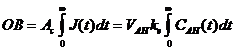(1)

where Ac is the area of the cornea, J(t) is the flux of drug across the cornea, VAH is the aqueous humor volume, ke is the aqueous humor elimination rate, and CAH(t) is the aqueous humor drug concentration3.  Using a theorem for the total mass-transport, eq 1 can be rewritten as follows: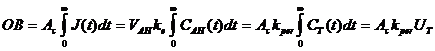(2)

where UT =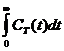, CT (t) is the drug concentration in the tear film, and kper is the corneal permeability coefficient: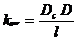(3)

where Dc is the distribution coefficient and D is the diffusion coefficient for drug in tissue, and l is the thickness of the cornea. We assume a uniform drug concentration throughout the entire precorneal fluid volume and a lack of drug metabolism.  Eq 2 predicts bioavailability inside the eye to be based on the time course of the tear film concentration outside the eye.

Thus it is necessary to calculate CT (t) and evaluate its integral over time, calculating an exact transient solution rather than the quasi-steady state solution, as was done previously. The time-dependent equation for CT (t) must take into account the mass diffusion transport across corneal and conjunctival membranes, the loss of excess fluid due to dosing volume, and the normal tear turnover rate.  As in the previous paper, the tear film volume (i.e., the combined volumes of the initial together with the excess dose volume) is given by2: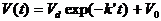(4)

where Vd is the drop volume in microliters, k' is the rate constant for removing the excess drop volume of fluid, and V0 is the natural volume of the tears. k' (per Ref 2) is given by k' = 0.25 + .0113Vd in units of per minute.

The mass balance equation governing the drug content of the tear film after dosing is as follows: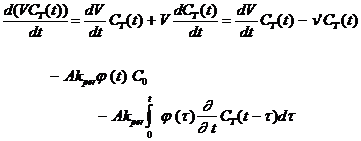(5)

where A is the total tissue area available for exposure to tears in the precorneal area and

where j(t), (the drug flux entering the corneal/conjunctival membranes when generated by a unit step in the drug concentration in the tear film), is given by: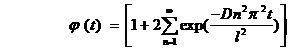(6)

That is, we account for the mass balance of drug in the tear film as it is depleted by the three factors of 1) drainage of excess tear fluid volume (from eq 4), as represented by the first term on the right hand side of (5)), 2) the natural turnover of the tear reservoir due to production of tears (the term v' CT (t), where n‘ is the tear turnover rate in units of volume/time, as represented by the second term on the right hand side of (5)), and 3) the loss of drug by transient diffusion through ocular tissue membranes (the last two terms in eq 5).  It is, of course, assumed that the ocular tissues are uniform as far as drug diffusion is concerned.   The term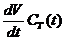cancels out on both sides.  The second-to-last term on the right hand side of equation (5) is equivalent to the solution to the problem of a drug-free membrane having diffusion coefficient D, thickness l and area A placed against a donor solution of constant drug concentration C0 at time zero.  The final term on the right hadn side of equation (5) arises from applying Duhamel’s theorem to the previously mentioned second-to-last term to account for the changes in the drug concentration of the tear film with time.  The value of the area A is taken to be one third of the total corneal and conjunctival area as deduced from the fit to eq (10) of reference 1.

Thus, the differential equation we wish to solve is: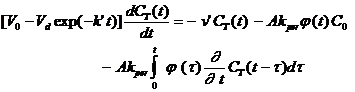(7)

The solution to this differential equation would need to be integrated over time to obtain the UT term noted in eq 2 above.  However, if use is made of a standard theorem for Laplace transforms, there is no need to solve the differential equation. The Laplace transform of  CT(t), evaluated at the zero value of the transform variable, yields the integral of interest:

Lim  s ® 0 C  (s) =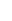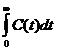(8)

Thus, the basic procedure is to take the Laplace transform of eq 7, and then take the limit of the Laplace transform of CT(t) as s approaches zero. The Laplace transform of eq 7 is as shown below: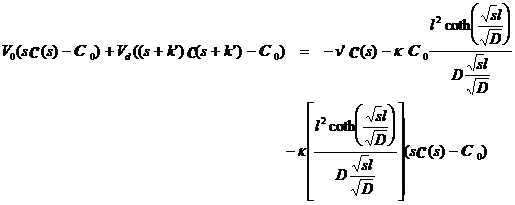(9)

where s is the transformed variable, C (s) is the Laplace transform of C(t), and k is equal to Akper.  The complicated coth terms on the r.h.s. of eq 9 arise from the Laplace transformed solution to the time-dependent membrane diffusion problem, with a unit step concentration applied to the donor surface (see Appendix 1).

The value of C (s) is therefore given by: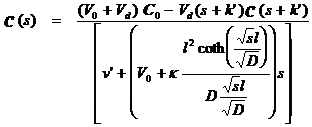(10)

The above formula can be iterated to obtain an infinite series for C (s).  Per the definition of UT in equation (3) together with equation (8), a series expression for UT can be obtained by setting s = 0 in this infinite series for C (s) :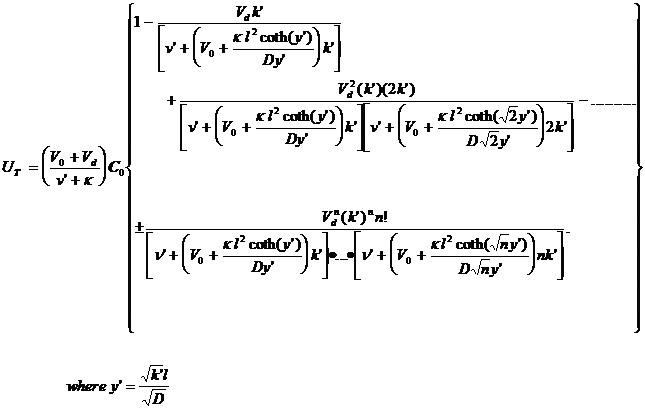(11)

Because C (s) is a decreasing function of s when s is real, the series will be convergent when Vd/V0 £ 1.  When Vd/V0 > 1, the series is divergent; that is, each successive term alternates between a lower and higher value and where the magnitude of successive terms eventually becomes unbounded.  Nevertheless, the actual value of UT can be estimated in these cases, since it is alternately bracketed by succeeding partial sums.  This latter condition is of prime interest because with a typical V0 value of 7.5 - 8 microliters and typical dose volumes greater than 15 microliters, the ratio Vd/V0 is almost always significantly greater than 1.

The variable UT (Vd/V0) can be normalized by dividing it by UT (0).  In this way, a comparison can be made between the bioavailability of a given dose volume to the bioavailability of a zero volume dose.  This ratio is readily obtained from the previous series as follows: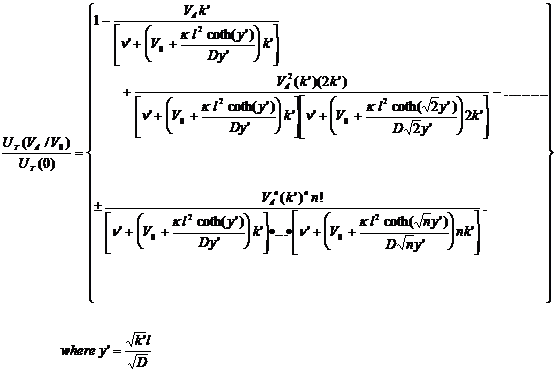(12)

A somewhat more useful expression is simply the inverse of the ratio on the left hand side of equation (12) which will be defined as R.  R is called the bioavailability improvement ratio of a zero volume dose to the dose volume in question, Vd.  It simply means the total dose required for a given dose volume, Vd, to supply a specified amount of drug to the target tissue divided by the total dose required for a zero volume dose to supply the same amount of drug to the target tissue.  To determine the estimates of the value of R, the UT(Vd/V0) to UT(0) ratio of equation (12) was equated to the series on the right and the resulting value of this ratio was inverted to obtain R.

When Vd/V0 is > 1 and in those cases where the distribution coefficient is high and the diffusion coefficient is low, the first few terms alternate closely around the actual  value for the ratio of equation (12).  When the reverse is true, (i.e., when the distribution coefficient is low and the diffusion coefficient is high), the series is more widely divergent, and a different mathematical technique is required to evaluate this series.  In Appendix 2, eq (12) is transformed into a more suitable form for the method described there.  It is important to note that although the new series brackets the ratio of equation (12) more closely when recast, it still is divergent whenever Vd/V0 > 1.  Never-the-less, the transformed series yields values for R which are accurate to at least 3 significant figures, (even when Vd = 30 microliters).  The reason this is so is that the successive partial sums differ from one another in the first few half dozen (or so) terms only in the third significant figure, at most.

Permeability coefficient and distribution coefficient data are available for a number of drugs. The permeability coefficient data are steady state across excised rabbit cornea, and the distribution coefficient is from octanol/buffer solution at pH of 7.65.  Calculations were made for the bioavailability improvement ratio for 30 microliters as compared to zero microliters for each of these drugs, using both the steady state expressions of the previous paper as well as the transient calculations of this paper.  A V0 of  7.5 microliters and a tear flow of 0.66 microliters/min. were used for these calculations (2).  The results are shown in table I.

The largest discrepancies between the transient and quasi-steady state results occur for drugs which have high distribution coefficients and average or low diffusion coefficients.  Under these circumstances, the drug flux is considerably higher than it would be under quasi-steady state assumptions, especially for large dose volumes.  Consequently, the steady state calculations will yield lower values for UT than the transient calculations, especially for large volumes.  This means that the actual dose required for a given specified amount of drug to the target tissue will be more for the quasi-steady state calculations. Thus, improvement ratios calculated using quasi-steady state assumptions would be artificially high. On the other hand, for those cases where the distribution coefficient is low and the diffusion coefficient relatively high, the lag time will be comparatively short, thus producing transient calculational results which are considerably closer to the steady state calculations.  Under these circumstances, the improvement ratio remains virtually unchanged from the previous steady-state prediction.

Figure 1 depicts a family of curves which show the improvement ratio as a function of the distribution coefficient.  Each of the six curves represents different (but constant) permeability coefficients.  When the distribution coefficient is small and when the permeability coefficient is held constant, this corresponds to large diffusion coefficients and short lag times, a situation in which the membrane diffusion very quickly comes to “quasi-steady state” equilibrium.  Alternatively, when the distribution coefficient is very large this corresponds to small diffusion coefficients and very long lag times.  Under these circumstances, the diffusion effects are equivalent to those sustained by a semi-infinite medium. Finally, there is a third transition region between the first two regions described, which corresponds to conditions where transient phenomena are important, but where the membrane thickness is still of some importance as well.  Each of these three regions is noted on the figure.  The steady state region corresponds to a ratio of permeability coefficient to distribution coefficient < 7.8 x 10-6 cm/sec; the transient region corresponds to the same ratio being > 780 x 10-6 cm/sec, and the transition region lies between these two values.  These two “break points” are somewhat arbitrary, and were determined by an examination of Figure 2, as discussed below.  An examination of Table 1 shows the various drugs listed in order of increasing permeability coefficient to distribution coefficient ratio, ranging from 0.355 to 52 x 10-6 cm/sec.  The transient - transition “break point” falls between metoprolol and nadolol such that of the drugs listed, 9 are in the transient region, 6 are in the transition region.  None of these drugs are actually in the steady state region, although atenolol and tobramycin are very close.

Figure 2 is depicts a family of curves of distribution coefficient versus diffusion coefficient, each of which represents a different value for the bioavailability improvement ratio. These curves asymptotically approach the quasi-steady state results, which are shown as vertical lines on the graph.  The straight line asymptotes shown on the upper left part portion of the Figure correspond to conditions where the tear film drug concentration decay due to partitioning is so rapid that the diffusion membranes themselves act like semi-infinite media of absorption.  Each of the curves, therefore, approaches either the quasi-steady state result (when the distribution coefficient is small) or the semi-infinite slab (when the distribution coefficient is very large).  The drugs noted in Table 1 are represented as single points on the graph.  The 30 microliter to zero volume improvement ratio is shown in parentheses underneath the points for each drug.  A curve fitting formula which yields bioavailability improvement results accurate to within 5% of the exact calculational results of the method described in the Appendix 2 was developed empirically.  It is as shown below: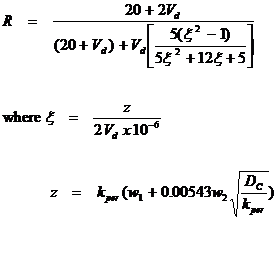(13)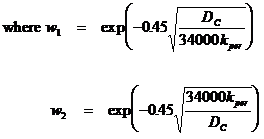It should be noted once again that Dc is the distribution coefficient (obtained as noted in Reference 8), Vd is the dose volume in microliters and kper is the permeability coefficient in cm/hr.  This formula can be used to determine the improvement ratio, R, of any one given dose volume to another by simply taking the ratio of the ratios.  For example, if the 30 microliter ratio (to zero volume) is 3.5 and the 5 microliter ratio (to zero volume) is 1.4, then the improvement ratio of 30 microliters to 5 microliters would be 3.5/1.4 = 2.5.

Application section

A study conducted with timolol shows that with a 25 microliter drop, the presence of a thickening agent (which (in effect) might produce close to a zero dose volume condition by essentially eliminating excess fluid drainage) resulted in an improvement ratio ranging between 1.4 and 2.8, depending on the specific tissue being examined.  The calculated improvement ratio for timolol, (based on a 25 microliter to zero volume reduction), is 1.84 which is well within the range of the reported experimental error.

It should be emphasized that this calculation does not take into account the generation of any additional liquid volume due to side effects of the drug (e.g., stinging for example).  It is possible, therefore, that changing a formulation from a drop to an insert or a viscous gel might increase the improvement ratio more than these calculations would predict, especially if the presence of the insert or gel were to reduce or eliminate the stinging effects of the drug as compared to when the drug is in a solution type formulation.

Conclusions

A more exact solution to transient effects of drug transport through the eye has been developed which shows that the quasi-steady state approach in effect yields an upper bound to the improvement in ocular bioavailability from reducing precorneal loss factors while maintaining constant dose of drug.  Both distribution coefficient and permeability coefficient in general play key roles in determining the volume improvement ratio. Bioavailability improvements calculated using quasi-steady state assumptions are artificially high when the drug distribution coefficient is high and the diffusion coefficient is low to average, but improve in accuracy as the diffusion coefficient increases or the distribution coefficient decreases.  As is apparent from an examination of Figure 1, the transient analysis can make a difference up to a complete elimination of the zero volume effect in the case where the distribution coefficient is large and the permeability coefficient is small (i.e., for large lipophilic molecules).  For the molecules shown in Table 1, the difference is not as much.

Acknowledgements

The authors gratefully acknowledge discussions and helpful suggestions obtained from Drs. E. Cooper, D. Hager, J. Lang, and G. Kasting.

Appendix 1

Laplace transform to the solution of the Unit Step Concentration applied to the donor side of a membrane

One starts with the standard diffusion equation, as follows: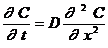(A1-1)

In addition, we apply the spatial boundary conditions of C = 0 at x = l, C = 1 at x = 0, and the initial condition and C = 0 for 0 < x £ l at t = 0.  Taking the Laplace transform of the above differential equation yields the following: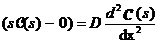(A1-2)

The general solution to the above equation can be expressed in terms of sinh and cosh functions as follows: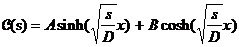(A1-3)

The Laplace transform of the solution at x = 0 (i.e., 1) is simply 1/s, while the Laplace transform of the solution at x = l is 0.  A and B are thereby determined and the final solution for C(s) is: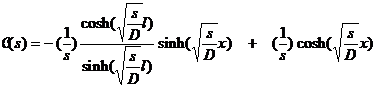(A1-4)

The quantity of interest is the flux of drug into the membrane, f(s), defined below: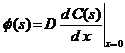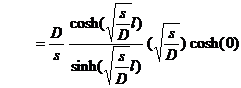(A1-5)

By making use of the distribution coefficient (Dc) and the definition of the permeability coefficient from eq (3), and also noting that the total diffusion loss of drug into the membrane must be the product of the area times the flux, we see that this loss term must be given by: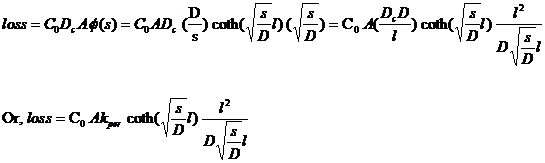(A1-6)

which is equivalent to the second loss term in equation 9 of the text.  The final loss term is obtained by making use of the convolution property of the Laplace Transform.

Appendix 2

If we let the function Z(x) be defined as follows: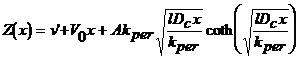(A2-1)

and the related sub-functions Zn(x):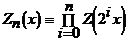(A2-2)

Using this approach, eq 12 of the text is transformed into eq A-3 below: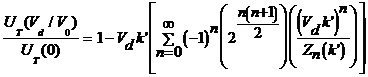(A2-3)

Series such as eq A2-3 often do not produce the desired limit with sufficient precision.  Techniques must be applied to extend the useful region of these expansions in cases where the series is known to be divergent.  The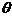transform of Brezinski was found to extract the antilimit* from the series with sufficient accuracy.

Thetransform is defined as follows for any nonconstant sequencea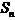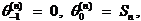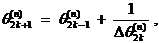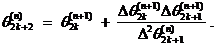Where the subscript represents the order of the transform, the superscript the term of the transformed sequence, and the difference operator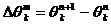.

This recursive transformb was originally derived from the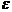transformc (p. 727 of reference 10).  Both of these transforms have been shown to have accelerative properties on large classes of convergent sequences,,, and antilimit extraction capabilities on certain classes of divergent sequences,.

The results presented in this paper were obtained by applying thetransform to the sequence of partial sums of the divergent series for UT(Vd/V0)/ UT(0).  When performing these calculations, the following practical considerations were taken into account:

80 bit floating point objects were used.d

For many values of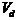,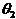was found to be sufficient.  For larger values of, however,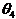was required.

The series for UT(Vd/V0)/ UT(0).   is a divergent series whenever Vd/V0 > 1.  The transform of this series is also a divergent series.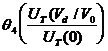will have a larger region of usefulness than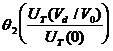which in turn will have a larger region of usefulness than the direct series for UT(Vd/V0)/ UT(0)**.

____________________

aIn this paper, the sequences are successive partial sums of the asymptotic series (that is,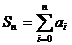).

bThis transform is more accurately described as a hierarchy of transforms.  The even ordered transforms provide the desired properties, the odd ordered transforms diverge.  The higher order transforms will typically accentuate the desired characteristics.

cFor information on the e transform, consult , , and 

dThe q transform, because it involves successive divisions by progressively smaller differences, typically

requires this much precision

*When the successive partial sums of a divergent series are known to oscillate above and below the value which the partial series approximates, when these sums contain convergent as well as divergent components and these convergent components dominate in the first few terms, the limit of these convergent components is called the “antilimit” of the divergent series.  By analogy, the antilimit of a divergent, oscillating series is the counterpart to the limit of a convergent, oscillating series in cases where the first few partial sums of the divergent series display successively smaller differences from one another.

**By a larger region of usefulness, we mean that Q4 produces a more accurate and (more useful) estimate of the antilimit than eqn. (12) itself produces in the region where the series diverges.  In this regard, the eqn. (12) estimates of the antilimits for several of the drugs noted in this paper were no better than 10 - 50% accurate for large Vd, in contrast to the 3 significant figure accuracy of the  Q4 series for these drugs.
References

1.  Keister, J. C., Cooper, E. R., Missel, P. J., Lang, J. C., and Hager, D. F. J. Pharm Sci. 1991, 80, 50-53

2.  Chrai, S.S., Patton, T.F., Mehta, A., Robinson, J.R.  J. Pharm Sci. 1973, 62, 1112-1121.

3.  Patton, T.F. in  Ophthalmic Drug Delivery Systems.; Robinson, J.R., Ed.; A.Ph.A.: Washington, 1980; Chapter 2

4.  Keister, J. C.,  J. Membr. Sci. 1986, 29, 333-344

5.  Crank, J.,  The Mathematics of Diffusion, Clarendon Press 1975, pg. 50, eq 4.22, where C2 is zero and C1 is C0 of eq 4.

6.  Carslaw, H.S. and Jaeger, J.C.,  Conduction of Heat in Solids, 2nd edition, Clarendon Press 1959, 30&31

7.  Nilsson, J.W.,  Electric Circuits, Addison Wesley 1986, 586-589

8.  Schoenwald, R. D., The Control of Drug Bioavailability from Ophthalmic Dosage Forms, Ch. 6 from Controlled Drug Bioavailability, Vol 3, Ed. Smolen & L. Ball, Wiley,  1985, 264-266

9.  Thermes, Florence, et. al, Bioadhesion: The Effect of Polyacrylic Acid on the Ocular Bioavailability of Timolol, Int. J. Pharm.,  81, 1992, 59-65

10.  Brezinski, C.,  Acceleration de suites a convergence logarithmique, C. R. Acad. Sc. Paris, 18 October 1971, 727-730

11.  Smith, D. A., Ford, W. F.,  Acceleration of Linear and Logarithmic Convergence, J. of Numerical Analysis, Fol 10, No. 2, April 1979, 223-240; see especially pg. 225

12. Ibid., Pgs. 225-226; pg 229; pgs 232-238

13.  Smith, D. A., Ford, W. F.,  Numerical Comparisons of Nonlinear Convergence Accelerators, Mathematics of Computation, , Vol 38, No. 158,  April 1982, pgs. 481-499; see especially pgs. 482-486

14.  Wynn. P.,  On the Convergence and Stability of the Epsilon Algorithm,  Siam J. of Numerical Analysis, Vol 3, No. 1, 1966, 91-122

15.  Wynn. P.,  On a Device for Computing the em(Sn) Transformation,  Mathematical Aids to Computation, Vol 10, 1956, 91-96

16.  Shanks, D.,  Non-linear Transformations of Divergent and Slowly Convergent Sequences,  J. of Mathematics and Physics, Vol 34, 1955, pgs. 1-42; see especially pgs. 16-21

17.  Reference 13, pgs. 486-488

Table 1.- 30 microliter to zero volume improvement ratio

 Drug kper x106,cm/sec DC (kper/Dc = D/l) x 106    cm/sec Bioavailability Enhancement Ratio Steady State Transient Transient/St State Bufuralol 72.4 204 0.355 1.51 1.07 0.709 Bevantolol 67.6 155 0.436 1.54 1.08 0.701 Acebutolol 0.85 1.58 0.538 3.86 3.05 0.790 Propranolol 47.6 41.7 1.141 1.68 1.17 0.696 Levobunolol 17.4 5.24 3.321 2.26 1.62 0.717 Cyclophosph. 11.3 2.4 4.708 2.56 1.96 0.766 Timolol 11.7 2.2 5.318 2.53 1.97 0.779 Oxyprenolol 27.5 4.9 5.612 1.97 1.53 0.777 Nadolol 1.0 0.151 6.623 3.83 3.65 0.953 Phenylephrine 0.94 0.100 9.400 3.85 3.73 0.969 Pilocarpine 17.4 1.7 10.235 2.26 1.91 0.845 Sotalol 1.6 0.056 10.256 3.69 3.66 0.992 Metoprolol 24 1.9 12.632 2.06 1.77 0.859 Atenolol 0.68 0.0302 22.517 3.91 3.91 1.000 Tobramycin 0.52 0.0100 52.000 3.98 3.98 1.000

Figure 1. - Family of curves for the therapeutic improvement ratio versus distribution coefficient.  Representative of the improvement in bioavailability which occurs upon reducing the dose volume from 30 mliters to zero at a constant dose.
Figure 2. - Family of curves of  distribution coefficient versus permeability coefficient, each representing a different therapeutic improvement ratio.  The therapeutic ratio represents the improvement in bioavailability which occurs upon reducing the dose volume from 30
mliters to zero at a constant dose.